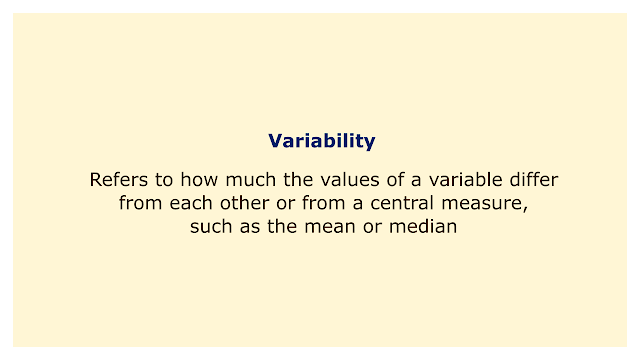# VariabilityImage: Moneybestpal.com

### Variability is a key concept in statistics and data analysis. It refers to how much the values of a variable differ from each other or from a central measure, such as the mean or median. There are numerous techniques to measure variation, including range, variance, standard deviation, interquartile range, and coefficient of variation.

Why is variability crucial? Variability aids in our comprehension of the reliability of our data and the degree of uncertainty in our findings. To determine the average height of a nation's population, for instance, we would need to gather a sample of heights from a representative sample of the population. Due to the wide range of individual heights, our sample will not be a perfect match for the population. We must thus measure the level of variability in our sample and how it affects our estimation of the population mean.

One method for doing this is to compute a confidence interval, which is a range of values that, with a certain degree of confidence, is likely to include the true population mean. The sample variability and sample size have an impact on the confidence interval. A confidence interval will be narrower with a higher sample size and lower sample variability, meaning more precision and less uncertainty.

Comparison of two or more data sets and testing for statistically significant differences between them are two additional ways to use variability. For instance, if we wish to compare the average heights of men and women in a nation, we must measure two samples of each gender's heights and figure out their means. However, because there is variation both within and between groups, we cannot just compare the means directly. In order to evaluate whether the difference between the means is greater than what would be predicted by chance, we must apply a statistical test, such as a t-test or an ANOVA, that accounts for the variability of both groups.

We may study the links and patterns in our data with the aid of variability. For instance, we can plot a collection of people's heights and weights on a scatterplot and search for patterns or clusters to see how height and weight are associated. However, since there might be variation in weight for a given height or vice versa, we also need to assess how strong and consistent the relationship is. As a result, we must employ a correlation coefficient, like Pearson's r or Spearman's rho, to measure how linearly related and how much they vary from one another two variables.

In conclusion, variability is a crucial element of statistics and data analysis that enables us to characterize, contrast, and draw conclusions from our data. We can make better decisions and infer more trustworthy inferences from our data by measuring and comprehending variability.
Tags